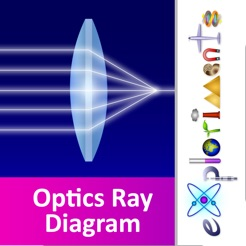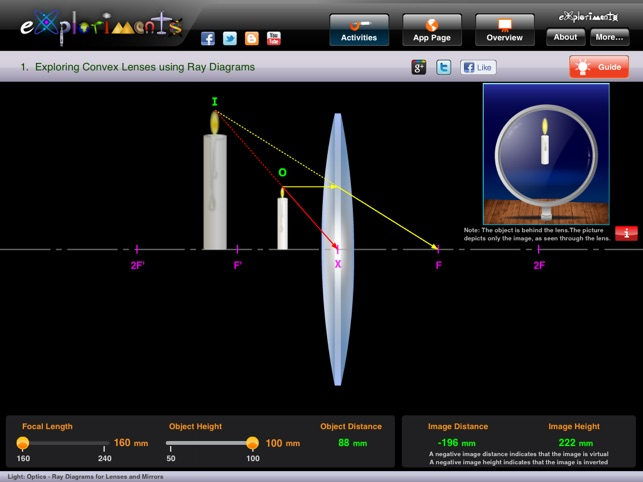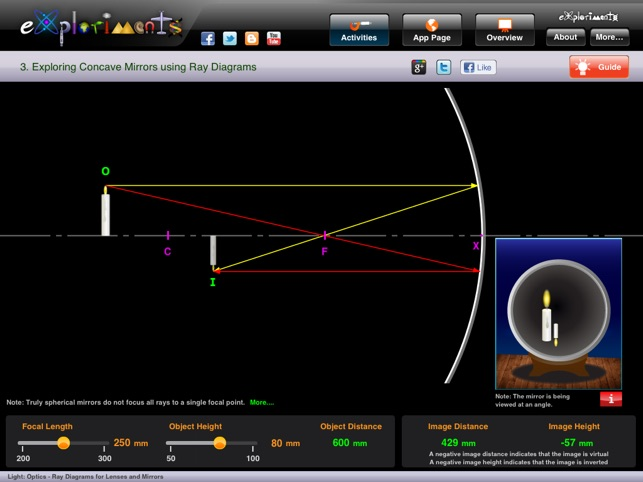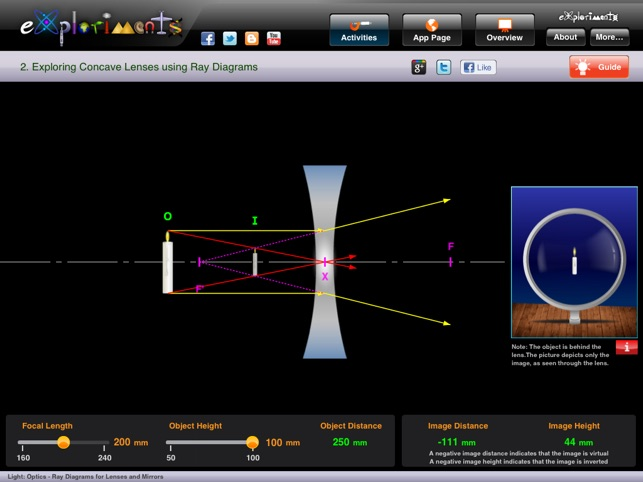••••Description

This Exploriments app has five activities that use highly interactive ray diagrams to introduce you to the basic concepts of optics. The app lets you explore reflective and refractive devices that are both converging and diverging in nature - the convex lens, concave mirror, convex mirror, concave lens and plane mirror. For each optical device, you can modify the focal length, object distance and object height and observe the effect it has on image formation, through a ray diagram format.

A ray diagram is a schematic that traces light rays from the object to the optical device – where they are either reflected or refracted - then on to the position where the image is created. This is a good method for understanding the size, position and orientation of an image. The app renders ray diagrams in real-time with every change in the object distance, focal length or object height.

The app describes the conventions that are needed to assign the proper signs to real, virtual, upright, inverted, diminished and magnified images. The app also helps you to apply the Gaussian Thin Lens Equation for solving numerical problems for given focal length and the different object and image properties. The accompanying theory content explains the characteristics of image formation in different optical devices with examples of real-world applications.

The Exploriments Series of interactive simulation-based tools makes conceptual learning in science an engaging and insightful experience. Visit www.exploriments.com to know more.

Activities:

Exploring Convex Lenses using Ray Diagrams
--- Analyze images characteristics by sections along the principal axis
--- Understand real, inverted images as formed by converging devices
--- Calculate and understand magnification and its relationship with focal length
--- Apply the Gaussian Equation to calculate image distance from focal length and object distance

Exploring Concave Lenses using Ray Diagrams
--- Understand the virtual upright images formed by this diverging device
--- Understand the practical applications of concave lenses based on the activities
--- Learn about negative focal length and image distance, apply to Gaussian Equation

Exploring Concave Mirrors using Ray Diagrams
--- Study image characteristics grouped by sections along the principal axis
--- Study the changes in image characteristic caused by changes in focal length
--- Distinguish between real and virtual image characteristics

Exploring Convex Mirrors using Ray Diagrams
--- Learn about the upright, virtual, diminished image as a characteristic of a diverging device
--- Learn about real life applications of a convex mirror
--- Learn the effect of object height and focal length on the characteristics of the image

Exploring a Plane Mirror using Ray Diagrams
--- Learn about virtual images that are the same size as the object
--- Understand the effect of changing object height on the characteristics of the image

--- An interactive simulation that uses ray diagrams to show real time changes with ability to change focal length, object distance and object height
--- A content Guide that takes you through Map, Explore and Discover phases

What’s New

Version 1.1

Bug fixes to support iOS 7.0

Information

Seller
IL&FS Education and Technology Services Ltd.
Size
15.5 MB
Category
Education
Compatibility

Requires iOS 4.3 or later. Compatible with iPad.

Languages

English

Age Rating
Rated 4+
Price
฿69.00

Supports

•